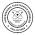### MATH EXERCISE 1 & 2

FIG 1.   Find the areas of the shaded regions.

FIG 2:    Find the area of the shaded region inside the trapezoid.

FIG 3:    Solve for the value of X.

FIG 4:    How long is the side of the square?

FIG 5:    Find the perimeter of the parallelogram.

FIG 6:    Find the area of the triangle.

FIG 7:    How long is the b2 of the trapezoid?

FIG 8:    Area = 108. Solve for X.

Figures below. Click image to enlarge.

1.Sir ask ko lng kung pano po naging 45.6 ung sagot sa figure 5, kasi lumalabas po na sagot sakin 48.92 pakicheck nmn po kung mali ako.. Thanks po!

1.45.6 is correct. try mo ulit esolve.

2.the longer side is 84/5=16.8; so P=2L+ 2W = 2(16.8) + 2(6) = 45.6

3.di po ba 5 yung h instead of 6? bale 2(16.8)+2(5)=43.6 or baka nalilito lang ako...